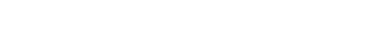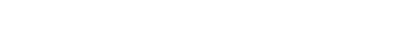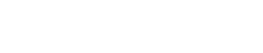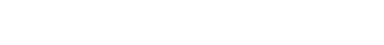# What Is the Binomial Theorem?

What is the Binomial Theorem? This algebraic tool is perhaps one of the most useful and powerful methods for dealing with polynomials! The Binomial Theorem shows how to expand any whole number power of a binomial — that is, (x + y)n — without having to multiply everything out the long way.This square represents the identity (a + b)2 = a2 + 2ab + b2 geometrically. The Binomial Theorem shows how to expand any power.

The Binomial Theorem is an important topic within the High School Algebra curriculum (Arithmetic with Polynomials and Rational Expressions HSA-APR.C.5). It also plays a significant role in college mathematics courses, such as Calculus, Discrete Mathematics, Statistics, as well as certain applications in Computer Science.

So, without further ado, let’s take a look at the Binomial Theorem!

## The Binomial Theorem

Suppose n is any natural number. ThenWhile the formula may look intimidating at first, there’s nothing to be afraid of. Let’s break it down!

The left side, (x + y)n, is an arbitrary binomial, x + y, raised to the power n. As you may recall from Algebra, a binomial is simply an algebraic expression having two terms.

The right side is the formula for expanding (x + y)n.

• It’s a sum (that’s what the “sigma” Σ symbol means) of certain kinds of terms. The second line of the formula shows how the sum expands explicitly.
• Each term begins with a number called a binomial coefficient. I’ve used one kind of notation in this formula, but you may have seen others.Don’t worry too much if you don’t understand the formula… there are other ways to find these numbers! (For more about the binomial coefficients, you might want to start here: Binomial Coefficients.)

• The next factors in each term are powers of x and y. Notice that the two powers always add up to n. That is, (nk) + k = n. This will be important as you work out problems.

### A Quick Example

Remember the rule, (a + b)2 = a2 + 2ab + b2? Well, we can work this out now using the Binomial Theorem together with Pascal’s Triangle.

With n = 2, x = a, and y = b in the formula, we find:The values of the binomial coefficients can be found using a Calculator (using the “nCr” function), or by Pascal’s Triangle.

You may be wondering why we used the Theorem in this case, since we already know a quick way to handle this situation, FOIL multiplication:

(a + b)2 = (a + b)(a + b) = a2 + ab + ba + b2 = a2 + 2ab + b2

That’s all well and good for squaring (n = 2) and cubing (n = 3) binomials. But what about the 5th power, or the 19th, and so on? Multiplying out by hand would be way too time-consuming and error-prone for large exponents without the Binomial Theorem.

### More About the Binomial Coefficients: Pascal’s Triangle

There is an amazing mathematical tool called Pascal’s Triangle, which easily generates all of the binomial coefficients!

Go get a blank page of notebook paper. Go on… I’ll wait. Now, on the top line of your paper, in the center, write a single “1.” Then write “1”s in two diagonals extending below your first “1” to the left and to the right. You can go down the page as far as you want to, but I stopped after seven more lines. Label each row, starting at the top with “n = 0,” n = 1,” etc.The beginning of Pascal’s Triangle

Next, starting at row n = 2 (which is actually the 3rd row!), the number that should fill in the blank space is found by adding the two numbers immediately above-left and above-right. That is, 1 + 1 = 2. Now your row n = 2 should look like:

1   2   1

Continue in this way down the triangle, always adding the two numbers above-left and above-right to generate the next number.

I’ve included arrows to show how each number is built from those above it:Pascal’s Triangle, complete up to row n = 7

The reason we care about Pascal’s Triangle is that its entries are exactly the values of the binomial coefficients, as long as you start counting at n = 0 down the rows and k = 0 across the diagonals.Interpreting Pascal’s Triangle as an array of binomial coefficients

So for example, look at row n = 4. The numbers are: 1  4  6  4  1. This means that:## Example Problems

Let’s do a few examples.

### Example 1: Nice and Easy

Expand: (3x + 2)3

#### Solution

You could do this one by multiplying everything out…However, let’s see how the Binomial Theorem works in this case.

First identify the power, n = 3. Then find the appropriate row in Pascal’s Triangle.

1   3   3   1

These numbers are the binomial coefficients for this example. Make sure you can spot where we use them in the computation below.

Also note that the original terms of the binomial are 3x and 2. Those items will replace x and y, respectively, in the formula.### Example 2: A Little Harder

Expand: (2m – 5p2)5

#### Solution

Again, look for the correct row of Pascal’s Triangle. The power is n = 5.

1   5   10   10   5   1

You must also rewrite the subtraction as addition in order to properly use the formula.

(2m — 5p2)5 = (2m + (–5p2))5

Now with x = 2m, and y = –5p2, we can proceed. (Note, this problem is not a good candidate for just multiplying out, as there would be five factors to deal with!)

Expand using the Binomial Theorem (binomial coefficients displayed in red in the second line). Be careful as you work out the exponents! (For a quick refresher on the rules of exponents, you might try: Quick Tips on Using the Exponent Rules.)As you can see, the solutions tend to get very large as the power n gets larger. Sometimes, however, a problem may ask only for a single term of a complicated expression. The Binomial Theorem can be used to find just that one term without having to work out the expression completely!

### Example 3: A Single Term

Find the degree 9 term of (4x3 + 1)6.

#### Solution

We can avoid working out the entire expression, by identifying which value of k corresponds to what’s being asked.Here, we need the exponent on x to be 9. According to the formula, the exponent of term k would be:

3(6 — k) = 18 — 3k

Setting that equal to 9 and solving, we find that k = 3.

Thus, the appropriate term is:## Author

•Shaun earned his Ph. D. in mathematics from The Ohio State University in 2008 (Go Bucks!!). He received his BA in Mathematics with a minor in computer science from Oberlin College in 2002. In addition, Shaun earned a B. Mus. from the Oberlin Conservatory in the same year, with a major in music composition. Shaun still loves music -- almost as much as math! -- and he (thinks he) can play piano, guitar, and bass. Shaun has taught and tutored students in mathematics for about a decade, and hopes his experience can help you to succeed!